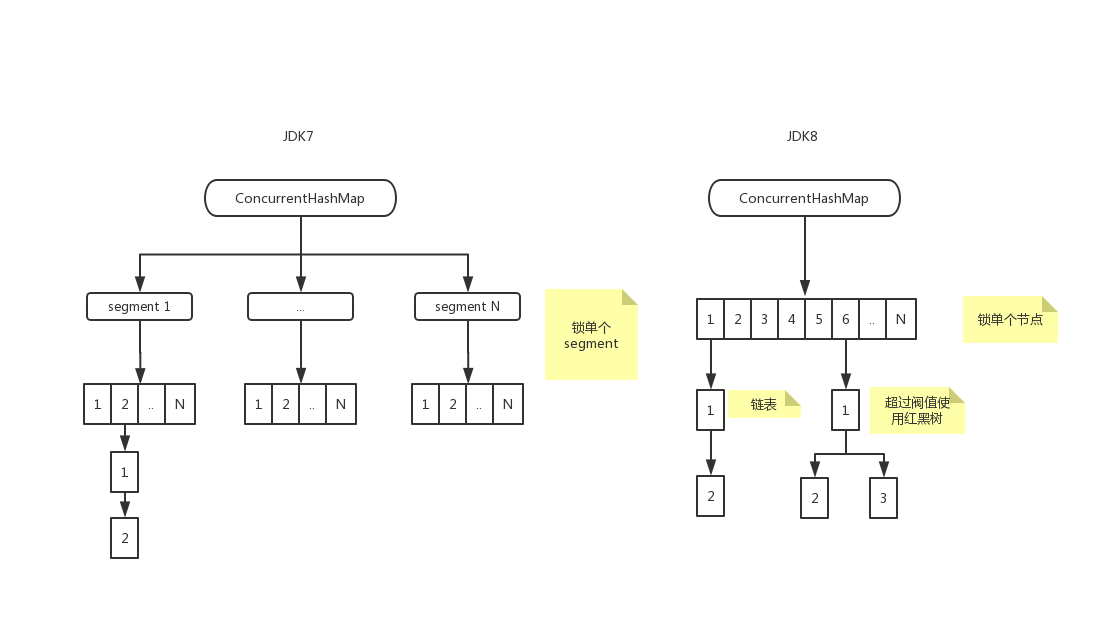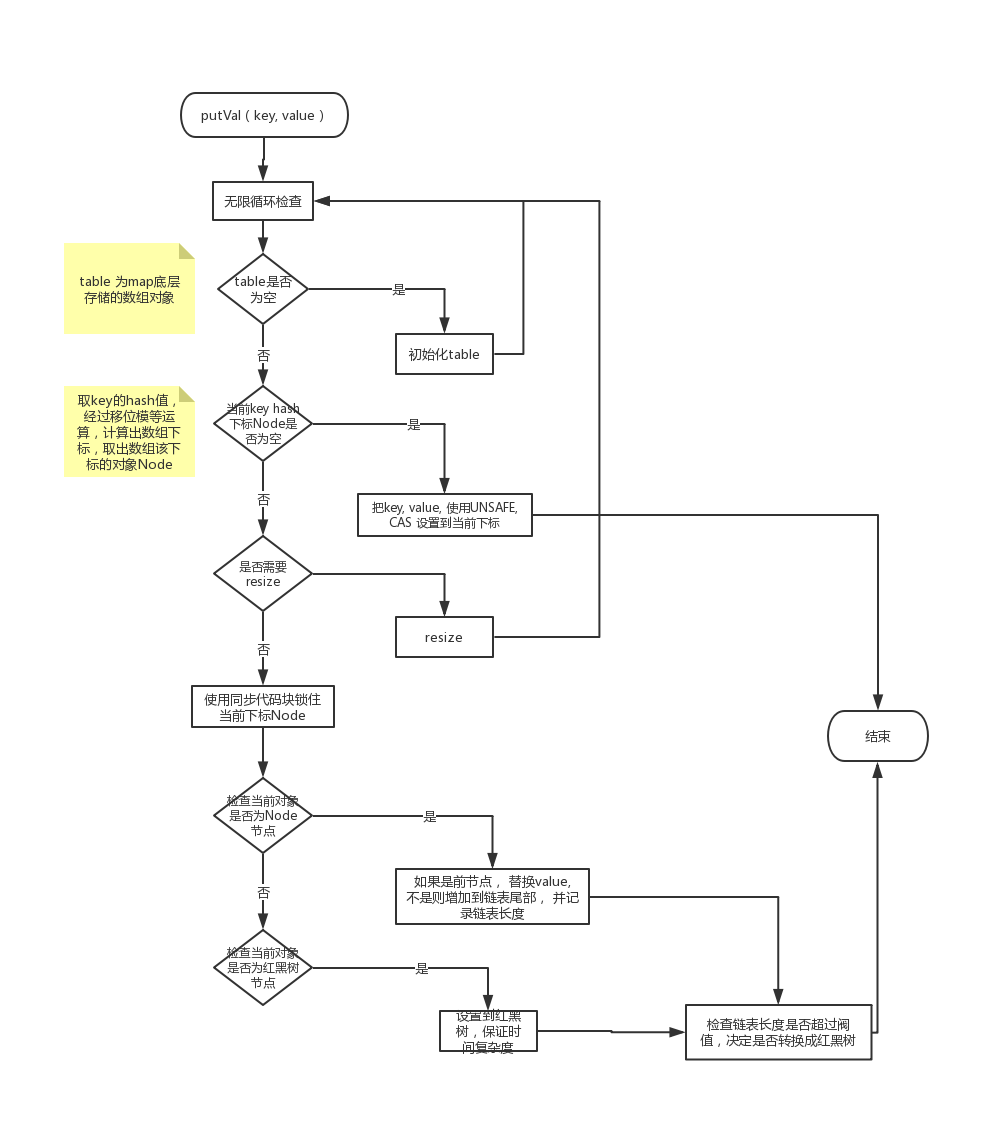# jdk8 ConcurrentHashMap 源码解析

2019/09/18 16:55

## jdk7 和 jdk8 的差异

jdk7 使用 ReentrantLock + segment + hashentry + unsafe
jdk8 使用 Synchronized + CAS + Node + NodeTree + Unsafe## put 方法

1. 检查table 是否初始， 没有的话初始化table，重新循环
2. 根据hash值模运算，计算出数组下标， 取出数组下标所在的值，如果值是null， 则用CAS设置到该下标处， 如果设置成功结束， 如果设置失败（失败原因可能是其它线程设置该下标的值） 重新循环
3. 待定
4. 如果当前下标的值不为空，进入同步代码块
1. 再次检查当前下标的值是否有改变，有改变结束当前，重新循环， 没有改变且是链表情况，逻辑比较好理解取出下标的值， 比较key 是否相当， 相等则设置新值， 不相等挂载链表， 同时记录链表长度
2. 如果是红黑树，则把值设置到红黑树（红黑树这里不做展开）
3. 根据链表长度，判断是否需要转换成红黑树， 默认阀值是8

## 上图更清晰## 源码（关键部分加了注释）

final V putVal(K key, V value, boolean onlyIfAbsent) {
if (key == null || value == null) throw new NullPointerException();
int hash = spread(key.hashCode());
int binCount = 0;
for (Node<K,V>[] tab = table;;) {
Node<K,V> f; int n, i, fh;
// 如果table为空， 初始化table, 详见下面
if (tab == null || (n = tab.length) == 0)
tab = initTable();
// 判断当前hash 的位置有没有值，没有值， 直接使使cas 无阻塞设置
else if ((f = tabAt(tab, i = (n - 1) & hash)) == null) {
if (casTabAt(tab, i, null,
new Node<K,V>(hash, key, value, null)))
break;                   // no lock when adding to empty bin
}
else if ((fh = f.hash) == MOVED)
tab = helpTransfer(tab, f);
else {
V oldVal = null;
// 只是锁住单个对象， 锁粒度更小
synchronized (f) {
// 再次检查是否有变更
if (tabAt(tab, i) == f) {
// 如果这个节点hash 值不为0， 意思是当前节点为普通节点的时候， 这里应该比较容易理解， 比较hash 值， key equals 是否相等， 如果hash 冲突就添加链表， 记录链表长度(binCount)，之后会根据长度调整， 是否使用红黑树代替链表
if (fh >= 0) {
binCount = 1;
for (Node<K,V> e = f;; ++binCount) {
K ek;
if (e.hash == hash &&
((ek = e.key) == key ||
(ek != null && key.equals(ek)))) {
oldVal = e.val;
if (!onlyIfAbsent)
e.val = value;
break;
}
Node<K,V> pred = e;
if ((e = e.next) == null) {
pred.next = new Node<K,V>(hash, key,
value, null);
break;
}
}
}
// 如果已经是树结构， 就按照树的结构来了
else if (f instanceof TreeBin) {
Node<K,V> p;
binCount = 2;
if ((p = ((TreeBin<K,V>)f).putTreeVal(hash, key,
value)) != null) {
oldVal = p.val;
if (!onlyIfAbsent)
p.val = value;
}
}
}
}
// 检查说阀值，默认是8， 超过会转换成树
if (binCount != 0) {
if (binCount >= TREEIFY_THRESHOLD)
treeifyBin(tab, i);
if (oldVal != null)
return oldVal;
break;
}
}
}
return null;
}


## get 方法(注释说明)

get 方法相对简洁很多， 主要逻辑已经put方法中处理

public V get(Object key) {
Node<K,V>[] tab; Node<K,V> e, p; int n, eh; K ek;
int h = spread(key.hashCode());
if ((tab = table) != null && (n = tab.length) > 0 &&
(e = tabAt(tab, (n - 1) & h)) != null) {
if ((eh = e.hash) == h) {
if ((ek = e.key) == key || (ek != null && key.equals(ek)))
return e.val;
}
// node 是红黑树时，查找对应节点
else if (eh < 0)
return (p = e.find(h, key)) != null ? p.val : null;

// 为链表时， 循环找出对应节点
while ((e = e.next) != null) {
if (e.hash == h &&
((ek = e.key) == key || (ek != null && key.equals(ek))))
return e.val;
}
}
return null;
}


## 初始化map底层数组 table（选读）

1. Unsafe

1. CAS

CAS，Compare and Swap即比较并交换，设计并发算法时常用到的一种技术，java.util.concurrent包全完建立在CAS之上，没有CAS也就没有此包，可见CAS的重要性。

1. 源码

private final Node<K,V>[] initTable() {
Node<K,V>[] tab; int sc;
while ((tab = table) == null || tab.length == 0) {
// sizeCtl: table 初始化和resize的标志位，表初始化和调整大小控件。当为负值时，将初始化或调整表的大小
if ((sc = sizeCtl) < 0)
// 如果是-1 表示正在初始化或者调整大小， 这时放弃cpu使用， 进行下一次循环检查
Thread.yield(); // lost initialization race; just spin
// 设置SIZECTL为-1，设置成功开始初始化， 不成功继续循环。
// compareAndSwapInt 非阻塞同步原语： arg0, arg1, arg2, arg3 分别为对象实例，目标对象属性，当前预期值，要设的值, 设置成功返回 true, 失败 false
else if (U.compareAndSwapInt(this, SIZECTL, sc, -1)) {
try {
if ((tab = table) == null || tab.length == 0) {
int n = (sc > 0) ? sc : DEFAULT_CAPACITY;
@SuppressWarnings("unchecked")
Node<K,V>[] nt = (Node<K,V>[])new Node<?,?>[n];
table = tab = nt;
sc = n - (n >>> 2);
}
} finally {
sizeCtl = sc;
}
break;
}
}
return tab;
}


## 总结

1. 用 Synchronized + CAS + Node + NodeTree 代替 Segment ，只有在hash 冲突， 或者修改已经值的时候才去加锁， 锁的粒度更小，大幅减少阻塞

2. 链表节点数量大于8时，会将链表转化为红黑树进行存储，查询时间复杂度从O(n)，变成遍历红黑树O(logN)。### 作者的其它热门文章

0
0 收藏

0 评论
0 收藏
0